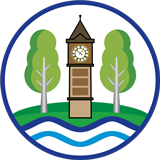# School Performance

2020 National Curriculum Assessments

In March 2020, the Secretary of State for Education announced that the 2019/20 national curriculum assessments would not take place due to the coronavirus (COVID-19) pandemic.
The following assessments were cancelled:

• end of key stage 1 and key stage 2 assessments (including tests and teacher assessment)
• phonics screening check
• multiplication tables check
• science sampling tests
• all statutory trialling

The department recognises this creates a gap in KS1 and KS2 attainment data for year 2 and year 6 pupils in 2020 and future progress measures that rely on that data will also be missing.

2019 KS2 SAT Results

Expected standard in Reading = 71%

Greater Depth in Reading = 27%

Expected standard in Maths = 81%

Greater Depth in Maths = 24%

Expected standard in Writing = 78%

Greater Depth in Writing = 7%

The number of children achieving the expected standard or above in reading, writing and maths (combined) = 63%

The number of children achieving a high level of attainment in reading, writing and maths (combined) = 3%

The average progress that children make in reading = -1.9

The average progress that children make in writing = -2.7

The average progress that children make in maths = -0.4

The average 'scaled score' in reading = 103

The average 'scaled score' in maths = 105

*****

2018 KS2 SAT Results

Expected standard in Reading = 72%

Greater Depth in Reading = 15%

Expected standard in Maths = 65%

Greater Depth in Maths = 20%

Expected standard in Writing = 72%

Greater Depth in Writing = 0%

The number of children achieving the expected standard or above in reading, writing and maths (combined) = 58%

The number of children achieving a high level of attainment in reading, writing and maths (combined) = 0%

The average progress that children make in reading = -2.7

The average progress that children make in writing = -2.6

The average progress that children make in maths = -0.2

The average 'scaled score' in reading = 102.0

The average 'scaled score' in maths = 103.4